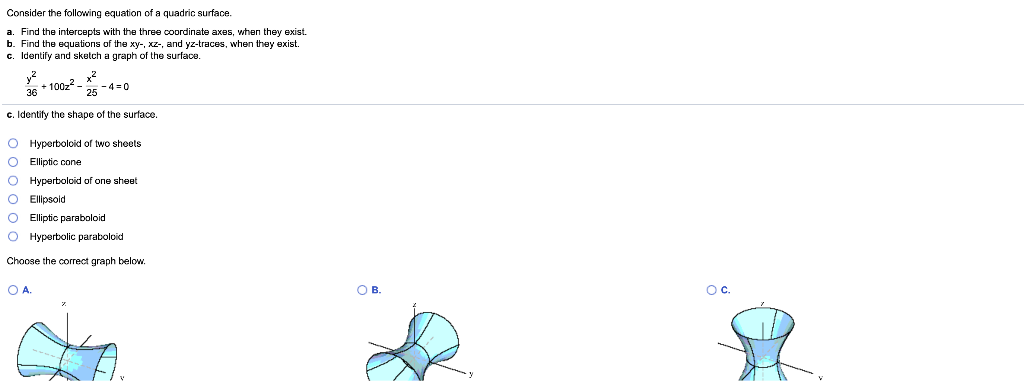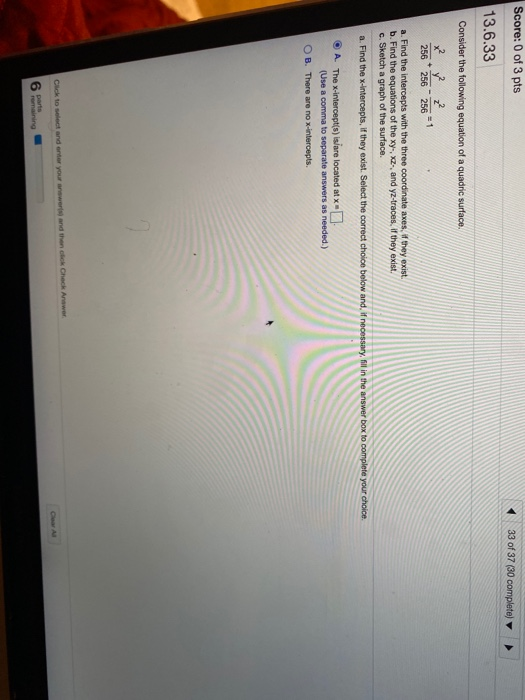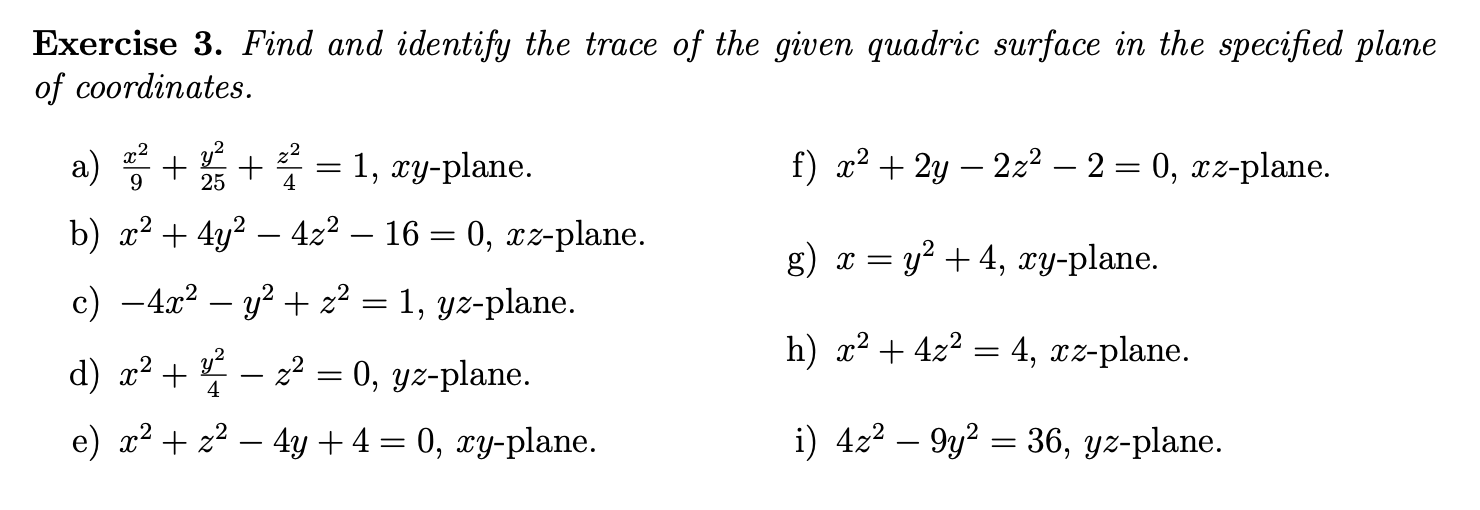Question

# Consider the following equation of a quadric surface. a. Find the intercepts with the three coordinate...Consider the following equation of a quadric surface. a. Find the intercepts with the three coordinate axes, when they exist. b. Find the equations of the xy-, xz-, and yz-traces, when they exist. c. Identify and sketch a graph of the surface. + 10022 - - 4-0 c. Identify the shape of the surface. Hyperboloid of two shoots 0 Elliptic cone Hyperboloid one sheet O Ellipsold O Elliptic paraboloid O Hyperbolic paraboloid Choose the correct graph below. OA ов. c. R

We need at least 10 more requests to produce the answer.

0 / 10 have requested this problem solution

The more requests, the faster the answer.

All students who have requested the answer will be notified once they are available.

#### Earn Coins

Coins can be redeemed for fabulous gifts.

Similar Homework Help Questions
• ### (a) Find and identify the traces of the quadric surface x2 + y2 ? z2 =...

(a) Find and identify the traces of the quadric surface x2 + y2 ? z2 = 16 given the plane. x = k Find the trace. y = k Find the trace. z = k Find the trace. Describe the surface from one of the graphs in the table. ellipsoid elliptic paraboloid hyperbolic paraboloid cone hyperboloid of one sheet hyperboloid of two sheets

• ### Score: 0 of 3 pts 33 of 37 (30 complete) 13.6.33 Consider the following equation of...Score: 0 of 3 pts 33 of 37 (30 complete) 13.6.33 Consider the following equation of a quadric surface. x2 2 2 256 256 256 a. Find the intercepts with the three coordinate axes. If they exist. b. Find the equations of the xy-, x2, and yz-traces, if they exist c. Sketch a graph of the surface. a. Find the x-intercepts, if they exist. Select the correct choice below and, if necessary, fil in the answer box to complete your...

• ### Consider the surface whose equation is z = -2(y+1)^2 + (x+1)^2 - 1 i) determine a...

Consider the surface whose equation is z = -2(y+1)^2 + (x+1)^2 - 1 i) determine a surface of the form (*) whose graph you can translate, rotate, or reflect to obtain the graph of the given surface. Provide the steps one would follow to manipulate the graph of your surface to get the graph of the given surface. Form could be Ellipsoid, Cone, Elliptic Paraboloid, Hyperboloid of One sheet, Hyperbolic paraboloid, and Hyperboloid of two sheets.

• ### Exercise 3. Find and identify the trace of the given quadric surface in the specified plane...Exercise 3. Find and identify the trace of the given quadric surface in the specified plane of coordinates. f) x2 + 2y – 2z2 – 2 = 0, xz-plane. g) x = y2 + 4, xy-plane. a) A + B + * = 1, xy-plane. b) x2 + 4y2 – 4z2 – 16 = 0, xz-plane. c) -4x2 - y2 + z2 = 1, yz-plane. d) x2 + – z2 = 0, yz-plane. e) x2 + x2 – 4y+4= 0,...

• ### (a) Find and identify the traces of the quadric surface x2 + y2 − z2 =...

(a) Find and identify the traces of the quadric surface x2 + y2 − z2 = 16 given the plane. x = k Find the trace. Identify the trace. circleellipse    hyperbolaparabola y = k Find the trace. Identify the trace. circleellipse    hyperbolaparabola z = k Find the trace. Identify the trace. circleellipse    hyperbolaparabola Describe the surface from one of the graphs in the table. ellipsoidelliptic paraboloid    hyperbolic paraboloidconehyperboloid of one sheethyperboloid of two sheets (b) If we change the equation in part (a) to...